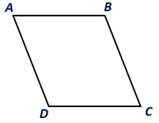Chapter 4.1, Problem 13E### Elementary Geometry for College St...

6th Edition
Daniel C. Alexander + 1 other
ISBN: 9781285195698

#### Solutions

Chapter
Section### Elementary Geometry for College St...

6th Edition
Daniel C. Alexander + 1 other
ISBN: 9781285195698
Textbook Problem
1 views

# Given that m ∠ A = 2 x 3 , and m ∠ C = x 2 + 20 , find themeasure of each angle of ▱ A B C D .To determine

To find:

The measure of each angle of ABCD.

Explanation

Given:

Given that Given that mA=2x3, and mC=x2+20, and the ABCD as shown below.

Corollary:

1. The opposite angles of a parallelogram are congruent.

2. Two consecutive angles of a parallelogram are supplementary.

In the given parallelogram ABCD, given that Given that mA=2x3, and mC=x2+20.

As per the corollary that the opposite angles of a parallelogram are congruent, the angles mA and mC are equal.

To find the value of x, substitute mA=2x3, and mC=x2+20 in mA=mC.

2x3=x2+20

2x3-x2=20

4x-3x6=20

x6=20

x=120

To find

### Still sussing out bartleby?

Check out a sample textbook solution.

See a sample solution

#### The Solution to Your Study Problems

Bartleby provides explanations to thousands of textbook problems written by our experts, many with advanced degrees!

Get Started